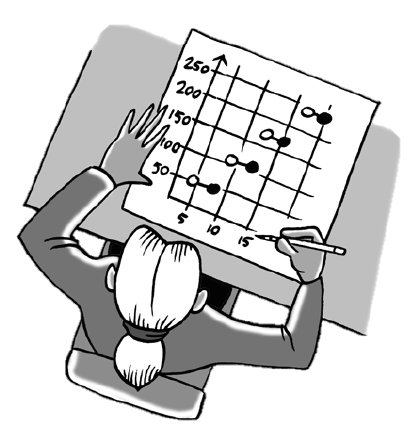### Home > INT3 > Chapter 3 > Lesson 3.1.4 > Problem3-65

3-65.

Jamillais moving to a new city. She researches the rates charged by the local utility company for water. She finds the listing of rate explanations below. She expects that her family may use up to $1000$ cubic feet of water each month.

• $\12.70$ monthly service fee

• First $300$ cubic feet of water used: $\3.90$ per $100$ cubic feet, or fraction thereof

• After the first $300$ cubic feet: $\5.20$ per $100$ cubic feet, or fraction thereof

1. Sketch a graph of the cost of Jamilla’s possible water usage in relationship to the cost during one month. Be sure to consider what the cost would be for partial units such as $220$ or $675$ cubic feet of water.

This will be a step graph. What is the cost if no water is used, but there is service?

When sketching this graph, note that the price jumps from $\3.90$ to $\5.20$ after $300$ cubic feet of water is used.

2. Is the graph for this situation a function? Why or why not?

For each $x$-value, is there exactly one $y$-value?

3. What are the domain and range of your graph?

Domain refers to the possible $x$-values, and range refers to the possible $y$-values.

4. If the monthly service fee is decreased to $\10.20$, how would that change the graph?

Would the overall price increase or decrease with this change?